SSC  >  Engineering Mechanics - 1

# Engineering Mechanics - 1

Test Description

## 20 Questions MCQ Test Mock Test Series for SSC JE Mechanical Engineering | Engineering Mechanics - 1

Engineering Mechanics - 1 for SSC 2023 is part of Mock Test Series for SSC JE Mechanical Engineering preparation. The Engineering Mechanics - 1 questions and answers have been prepared according to the SSC exam syllabus.The Engineering Mechanics - 1 MCQs are made for SSC 2023 Exam. Find important definitions, questions, notes, meanings, examples, exercises, MCQs and online tests for Engineering Mechanics - 1 below.
Solutions of Engineering Mechanics - 1 questions in English are available as part of our Mock Test Series for SSC JE Mechanical Engineering for SSC & Engineering Mechanics - 1 solutions in Hindi for Mock Test Series for SSC JE Mechanical Engineering course. Download more important topics, notes, lectures and mock test series for SSC Exam by signing up for free. Attempt Engineering Mechanics - 1 | 20 questions in 12 minutes | Mock test for SSC preparation | Free important questions MCQ to study Mock Test Series for SSC JE Mechanical Engineering for SSC Exam | Download free PDF with solutions
 1 Crore+ students have signed up on EduRev. Have you?
Engineering Mechanics - 1 - Question 1

### The resultant of two forces each equal to P and acting at right angles is:

Detailed Solution for Engineering Mechanics - 1 - Question 1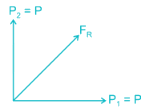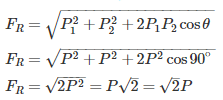Engineering Mechanics - 1 - Question 2

### Which of the following conditions do not change the effect of couple?

Detailed Solution for Engineering Mechanics - 1 - Question 2

Two parallel forces equal in magnitude and opposite in direction and separated by a definite distance are said to form a couple.

The translatory effect of a couple on the body is zero.

The only effect of a couple is a moment and this moment is same about any point, the effect of a couple is unchanged if:

(i) The couple is rotated through any plane

(ii) The couple is shifted to any other position

(iii) The couple is replaced by another pair of forces whose rotational effect is same

Engineering Mechanics - 1 - Question 3

### Two car A and B moves at 54 Km/hr in the same direction and the car B is 300 m ahead of car A. If the car A is accelerated at 6 m/s2 while car B continue to move with the same velocity, calculate the time taken by car A to overtake car B:

Detailed Solution for Engineering Mechanics - 1 - Question 3

uA = uB = 54 Km/hr = 15 m/s; aA = 6 m/s2, aB = 0

Car B is 300 m ahead of car A.

Let both move in time t sec.

Distance travelled by A in time t = 300 + Distance travelled by B in time t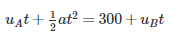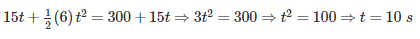Engineering Mechanics - 1 - Question 4

A couple is formed when:

Detailed Solution for Engineering Mechanics - 1 - Question 4

A couple consists of two parallel forces that are equal in magnitudeopposite in sense and do not share a line of action. It does not produce any translation, only rotation. The resultant force of a couple is zero. But, the resultant of a couple is not zero; it is a pure moment.

Engineering Mechanics - 1 - Question 5

A ball of mass 1 kg moving with the velocity of 2 m/s collide directly with another stationary ball of mass 2 kg and comes to rest after impact. The velocity of second baII after impact is:

Detailed Solution for Engineering Mechanics - 1 - Question 5

Conservation of linear  momentum: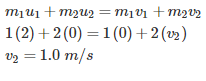Engineering Mechanics - 1 - Question 6

Concurrent force system is the system when:

Detailed Solution for Engineering Mechanics - 1 - Question 6

Collinear Forces: Line of action of all the forces act along the same line.

Coplanar parallel forces: All forces are parallel to each other and lie in a single plane

Coplanar concurrent forces: Line of action of all forces pass through a single point and forces lie in the same plane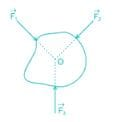Coplanar non-concurrent forces: All forces do not meet at a point, but lie in a single plane

Non-coplanar parallel forces: All the forces are parallel to each other, but not in same plane

Non-coplanar concurrent forces: All forces do not lie in the same plane, but their lines of action pass through a single point

Non-coplanar non-concurrent forces: All forces do not lie in the same plane and their lines of action do not pass through a single point

Engineering Mechanics - 1 - Question 7

Two rings of radius R and nR, made up of same material (linear mass density) have the ratio of moment of inertia about an axis passing through centre as 1 : 8. The value of n is

Detailed Solution for Engineering Mechanics - 1 - Question 7

For ring:

MOI about diametric axis: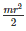MOI about polar axis: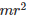Linear mass density: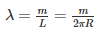m=λ×2πR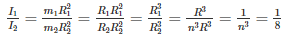n = 2

Engineering Mechanics - 1 - Question 8

A 13 m ladder is placed against a smooth vertical wall with its lower end 5 m from the wall. What should be the coefficient of friction between the ladder and floor so that it remains in equilibrium?

Detailed Solution for Engineering Mechanics - 1 - Question 8

Without knowing the weight of the ladder, coefficient of friction between the ladder and floor cannot be found.

Engineering Mechanics - 1 - Question 9

The torque acting on a body, rotating with angular acceleration of ‘α’ is given by which of the following relation?

Detailed Solution for Engineering Mechanics - 1 - Question 9

Ft=mat=mrα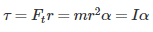Engineering Mechanics - 1 - Question 10

A 1.0 kg ball drops vertically onto the floor with a speed of 25 m/s. It rebounds with an initial speed of 10 m/s. The impulse action on the ball during contact will be

Detailed Solution for Engineering Mechanics - 1 - Question 10

Impulse = change in momentum

= mv- mv1

= 1 (10) - 1 (-25) = 35 N - s

Engineering Mechanics - 1 - Question 11

The area moment of inertia of a square of size 1 unit about is diagonal is

Detailed Solution for Engineering Mechanics - 1 - Question 11

For a square of side a, the area moment of inertia of square with respect to one diagonal is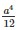Here a = 1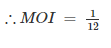Engineering Mechanics - 1 - Question 12

If tension in the cable supporting a lift moving downwards is half the tension when it is moving upwards, the acceleration of the lift is

Detailed Solution for Engineering Mechanics - 1 - Question 12

When lift is at rest: T = mg

When lift is accelerating upward: TU = mg + ma

When lift is accelerating downward: TD = mg - ma

TU = 2TD

mg + ma = 2mg - 2ma

a = g/3

Engineering Mechanics - 1 - Question 13

The moment of inertia of a square of side (a) about an axis though its center of gravity is

Detailed Solution for Engineering Mechanics - 1 - Question 13

Moment of inertia of a rectangle about an axis though its center of gravity is bd3/12 and of a square is a4/12.

Engineering Mechanics - 1 - Question 14

Moment of inertia of an area always least with respect to

Detailed Solution for Engineering Mechanics - 1 - Question 14

Mass distribution is minimum about centroidal axis, so moment of inertia of an area always least with respect to ​centroidal axis

Engineering Mechanics - 1 - Question 15

Which of the following is an example of a body undergoing translational equilibrium?

Detailed Solution for Engineering Mechanics - 1 - Question 15

An object is said to be in equilibrium when there is no external net force acting on it. When an object is in equilibrium, it does not accelerate. If it had a velocity, the velocity remains constant; if it was at rest, it remains at rest.

If all the forces acting on a particular object add up to zero and have no resultant force, then it’s in translational equilibrium. Examples would be a book resting on a bookshelf, or someone walking at a steady, constant speed.

An object that’s not rotating or doing so at a steady speed, the sum of the torques acting on it equaling zero, is at rotational equilibrium. Some examples of this are a Ferris wheel turning at a constant velocity, two children of equal weight balanced on either side of a seesaw, or the Earth rotating on its axis at a steady speed.

Engineering Mechanics - 1 - Question 16

A circular disc rolls down an inclined plane, the fraction of its total energy associated with its rotation is _____

Detailed Solution for Engineering Mechanics - 1 - Question 16

Total energy of the disc rolling down on an inclined plane is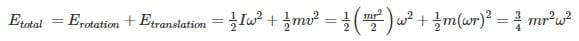Total rotational energy is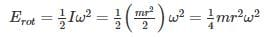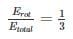Engineering Mechanics - 1 - Question 17

A body of mass 10 kg moving with a velocity of 1 m/s is acted upon by a force of 50 N for two seconds. The final velocity will be:

Detailed Solution for Engineering Mechanics - 1 - Question 17

Velocity = mass × acceleration

∴ 50 = 10 × a

⇒ a = 5 m/sec2

Velocity after 2 seconds,

v = u + at

= 1 + 5 × 2

= 11 m/sec

Engineering Mechanics - 1 - Question 18

A beam is fixed at one end and is vertically supported at the other end. What is the degree of statical indeterminacy?

Detailed Solution for Engineering Mechanics - 1 - Question 18

If the number of independent static equilibrium equations is not sufficient for solving all the external and internal forces in a system, then the system is said to be statically indeterminate.

Degree of static indeterminacy = Total no. of unknowns (External and internal) – Number of independent equations of equilibrium.

Now in this problem,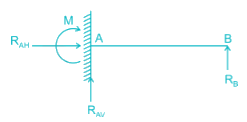No. of unknowns = 4 (RAH, RAV, M and RB)

No. of independent equations of equilibrium = 3 (∑H = 0, ∑V = 0, ∑M = 0)

∴ Degree of static indeterminacy - 4 - 3 = 1

Engineering Mechanics - 1 - Question 19

The angular motion of a disc is defined by the relation (θ = 3t + t3), where θ is in radians and t is in seconds. What will be the angular position after 2 seconds?

Detailed Solution for Engineering Mechanics - 1 - Question 19

Angular motion θ = 3t + t3

Motion covered in 2 seconds = 3 * 2 + 2= 14 rad. So the angular position after 2 seconds will be 14 rad.

Engineering Mechanics - 1 - Question 20

A car moving with uniform acceleration cover 450 m in a 5 second interval, and covers 700 m in the next 5 second interval. The acceleration of the car is

Detailed Solution for Engineering Mechanics - 1 - Question 20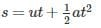at t = 5 sec, s = 450 m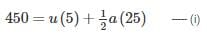at t = 10 sec

s = 450 + 700 = 1150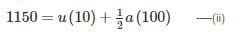Equation (ii) – Equation (i), we get

a = 10 m/s2

## Mock Test Series for SSC JE Mechanical Engineering

3 videos|1 docs|55 tests
 Use Code STAYHOME200 and get INR 200 additional OFF Use Coupon Code
Information about Engineering Mechanics - 1 Page
In this test you can find the Exam questions for Engineering Mechanics - 1 solved & explained in the simplest way possible. Besides giving Questions and answers for Engineering Mechanics - 1, EduRev gives you an ample number of Online tests for practice

## Mock Test Series for SSC JE Mechanical Engineering

3 videos|1 docs|55 tests